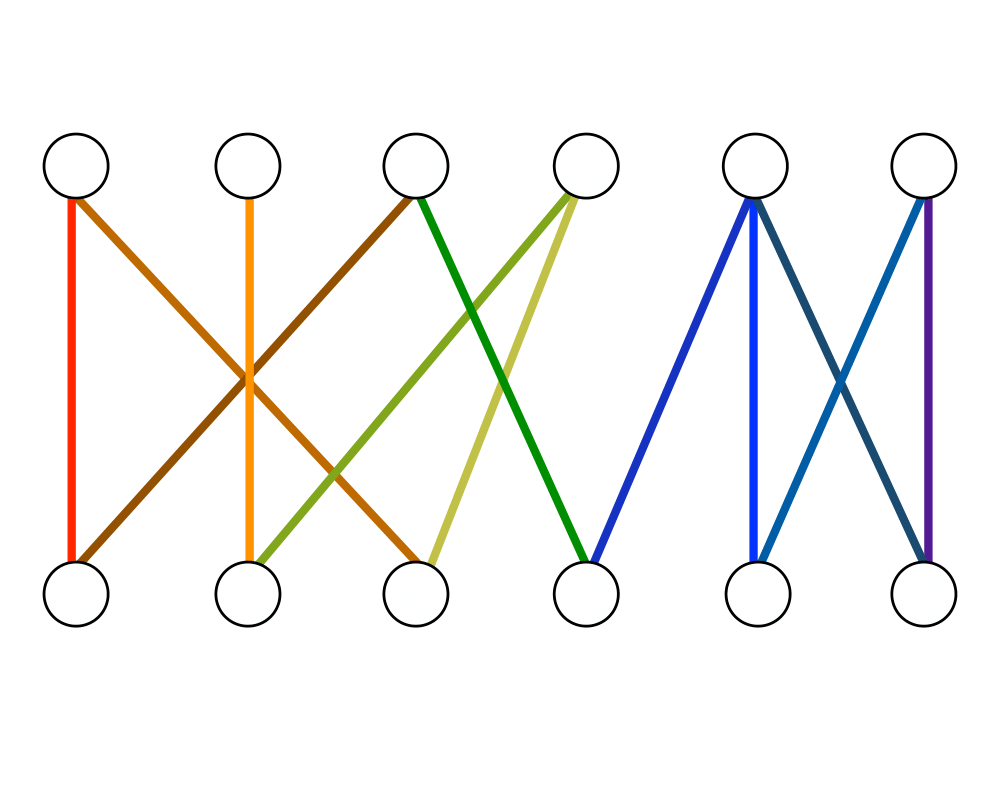# MatchingInputOutput

Input Description: A (weighted) graph $$G=(V,E)$$.
Problem: Find the largest size set of edges $$S \in E$$ such that each vertex in $$V$$ is incident to at most one edge of $$S$$.

Excerpt from The Algorithm Design Manual: Consider a set of employees, each of whom is capable of doing some subset of the tasks that must be performed. We seek to find an assignment of employees to tasks such that each task is assigned to a unique employee. Each mapping between an employee and a task they can handle defines an edge, so what we need is a set of edges with no employee or job in common, i.e. a matching.

Efficient algorithms for constructing matchings are based on constructing augmenting paths in graphs. Given a (partial) matching $$M$$ in a graph $$G$$, an augmenting path $$P$$ is a path of edges where every odd-numbered edge (including the first and last edge) is not in $$M$$, while every even-numbered edge is. Further, the first and last vertices must not be already in $$M$$. By deleting the even-numbered edges of $$P$$ from $$M$$ and replacing them with the odd-numbered edges of $$P$$, we enlarge the size of the matching by one edge. Berge's theorem states that a matching is maximum if and only if it does not contain any augmenting path. Therefore, we can construct maximum-cardinality matchings by searching for augmenting paths and stopping when none exist.

Go To Main Page